Choose Language Hide Translation Bar
Neural networks for regression lovers

Neural networks sound pretty fancy. Neural makes you think of brains. Which are complicated. But if you are familiar with linear regression, you have the building blocks needed to understand what a neural net does.

Neural networks (NNs) are generally classified under Supervised Machine Learning (although there are types of NNs that can do amazing things without labeled data as well #AutoEncoders). They take a set of inputs (a set of variables you want to use to predict something) and predict an outcome.

Which...sounds a lot like linear regression.

In linear regression, we take one or more inputs, and predict a continuous output by using the formula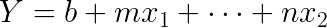Where Y is what you want to predict, and the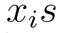are your inputs. For example, we might want to predict the amount of money you spend annually on video games based on your Age, Sex, Salary, and Rent.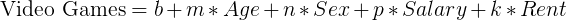Then we let the regression learn the values of the coefficients (this might seem like a silly way to say this, but stick with me).

We don’t tell the regression what the coefficients are, instead we tell it how to learn them. In the simple case, we often ask the regression to use the Least Squares method to learn what the optimal coefficients are.

In essence, we give the regression a structure for the model, and a method for learning the values in the model. Then, we set it on its way to learn.

When we build a neural network, we will also choose a structure and a method for learning, but they’ll often look a little different than the structure and method we use for regression.

### A Regression Example

STRUCTURE

Nodes. Neural networks use nodes as containers to hold values. These nodes have connections between them. When they’re drawn out graphically, they kinda look like a bunch of lollipops laid side by side.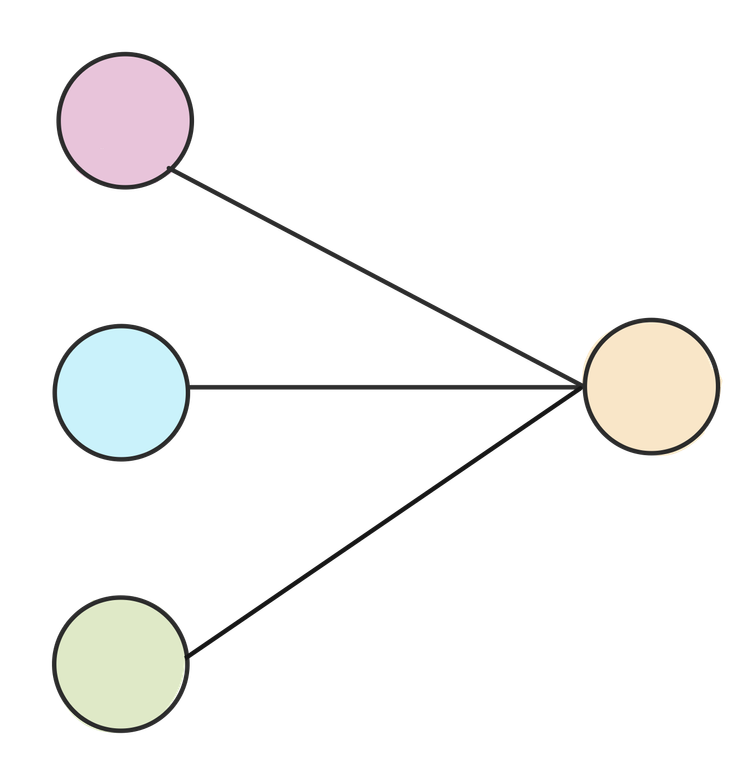The connections represent mathematical calculations that are applied to the values of one node as they use the connection to travel to the next.

If we wanted to build our video game regression as a neural network, we totally could! It’s structure would look like this: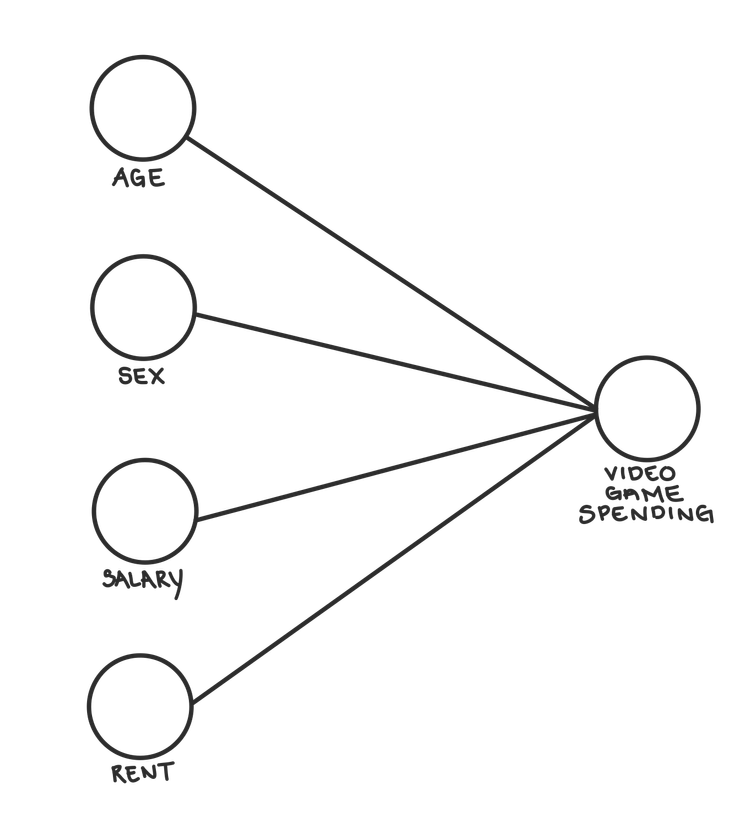Layers. Our video game NN has two layers: an input layer where we give the NN our predictor variables age, sex, salary, and rent, and an output layer where we get our prediction out of the NN.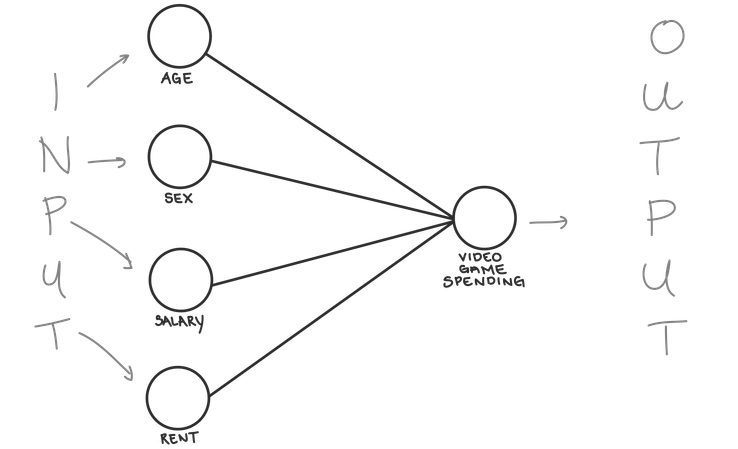The connections between the two layers contain weights, we can call them m, n, p and k, which we will apply to each variable as it goes from the input layer to the output layer. So age will get multiplied by m, sex by n, and so on, and all of these products will be added together in the output node.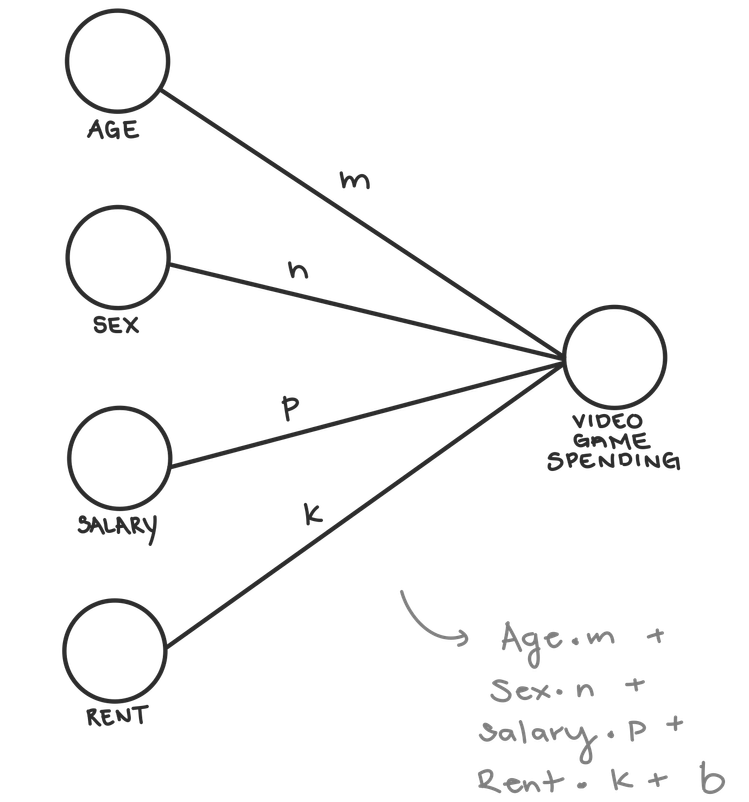Neural networks also add a bias (b) to to the value in the output node. The bias is just a scalar (a regular number like 1, -7, or 42).

Based on the neural net structure we built, the output node would give us a result based on this formula:Which is the same as the regression formula we saw above.

LEARNING METHOD

When we do a linear regression, we have to find/calculate the values of our coefficients (b,m,n,p, and k) after we specify the model structure. Often we use the Least Squares method to find our coefficients.

In short, Least Squares finds the coefficients that minimize the sum of squared errors between the model’s prediction, and actual data.

In math-y terms, we minimize this function: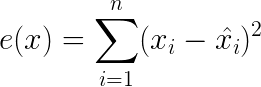Where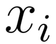is the actual value of a data point, and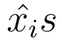is the model’s prediction for that data point.

Loss. If we felt like it, we could call this function our loss function. And that’s exactly what it’s called when working with neural networks. Loss functions provide us with a numerical measure of how well our model is doing. If loss is small, the predictions are pretty accurate. If it’s big, it’s not doing so hot. Our goal in building a good model is always to minimize our loss function (and therefore maximize model performance).

And while Mean Squared Error (the e(x) from least squares) is a good choice for many models, there are many other choices. Neural networks can have all kinds of structures, and different data/structures might benefit from other types of loss functions.

“Learning.” We know we want to minimize our loss function, and we have our data and the structure we used to build our neural net, but we actually need to *do* the minimization. This is where our neural net will start to deviate from our regression model.

In simpler regressions there’s a pretty easy and quick mathematical way to solve for our exact coefficients either using linear algebra, geometry, and regular algebra. But neural networks take a slightly different approach. Neural Networks often use iterative approaches to find values that minimize the loss function (and optimize our model). Essentially, we try to take a step closer to the “best” answer at each step in our iteration.

Many tools and tutorials use some form of Gradient Descent. I won’t spend a ton of time on the technical details of traditional Gradient Descent because it’s not what JMP uses, but the general idea is that there is a gradient  of the loss function (think a map with mountains and valleys) where higher points represent poorer model performance, and lower points represent good, even optimal model performance.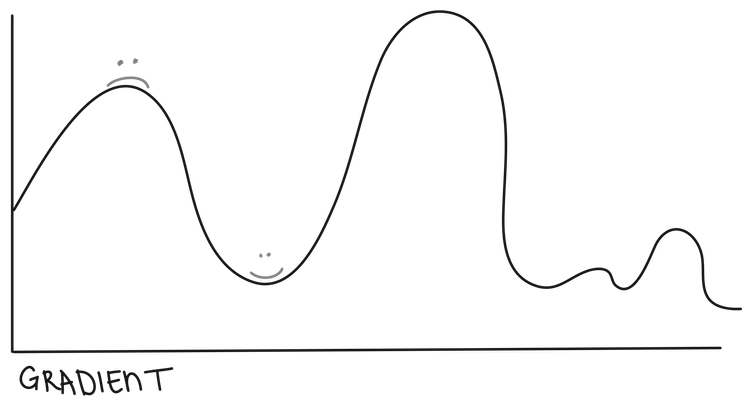You can imagine gradient descent as a hiker who is on this map trying to get to the lowest point possible. For each step they take, they look around and figure out which direction has the steepest descent and step in that direction. Eventually, they end up at a low point. Returning from our hiking analogy, the low point represents a set of parameters (like our coefficients and bias) where the loss function is minimized and therefore our model is doing pretty well!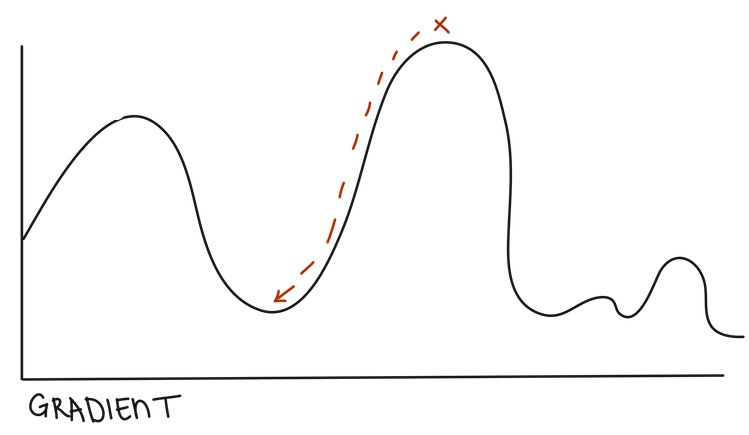In JMP, a slightly different technique is used to optimize a NN. Instead of using typical Gradient Descent methods, JMP uses something called the BFGS algorithm (that does not stand for Big Friendly GiantS, sorry to the Roald Dahl fans). The BFGS algorithm helps iteratively take steps to optimize the model, similarly to Gradient Descent, but uses different math to do so.

Instead of waiting till the algorithm finds an ultimate low point (one of the valleys in your gradient), JMP decides that a model is optimized by looking at a validation set (this is why JMP will require one, or will hold out a portion of your data for validation automatically, when  building a NN). The validation set consists of data that are NOT used to optimize the model.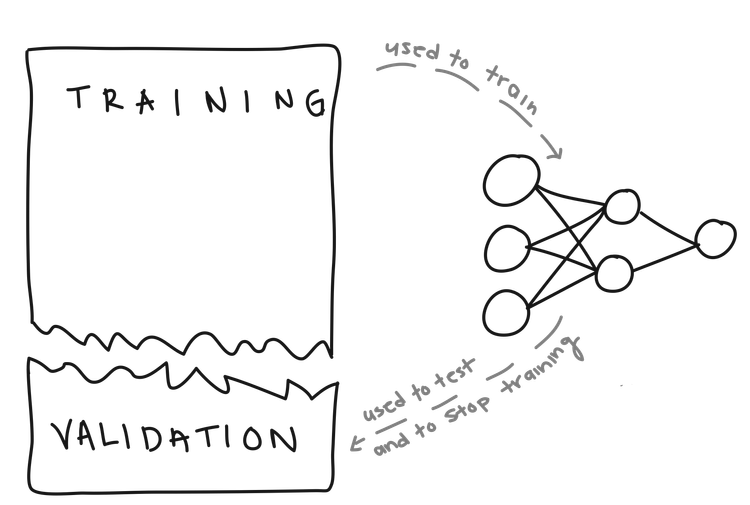JMP will test the current model on the validation set. Once taking more “steps” towards the low point doesn’t significantly improve the model’s performance on the validation set, JMP considers the model optimized. Testing the model on new data that wasn’t used to create the model helps us understand how the model we’re creating might perform on future data. This method is referred to as “Early stopping” because it let’s us stop taking steps towards the low point earlier. This often lets us optimize our model quickly.

No matter which method is used, once the model is considered sufficiently optimized, we now have all the estimates for the parameters in our Neural Network! We finally have a working model that we can use to predict something.

### A More Complex Structure

Now you know the basics of how neural networks are structured, and how they learn. But not all neural networks have to look like the regression one we did above. Neural networks allow us to be a lot more flexible when building their structure.

Multiple layers. One thing we can do with neural nets is add more layers. In our regression example, we had an input layer that was directly connected to our output layer. But we can insert an extra layer between them and connect each layer to the next.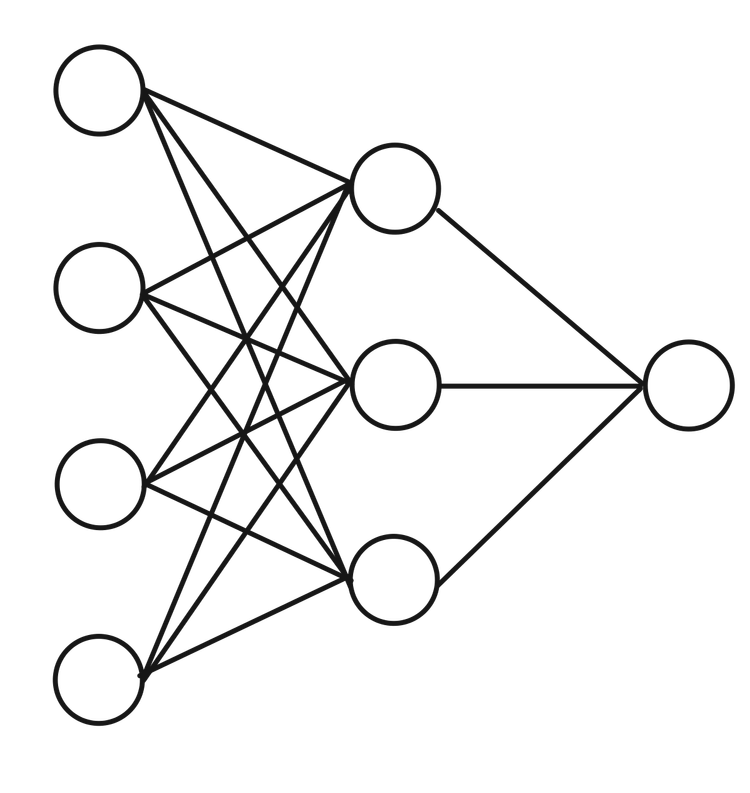This means that the input data will go through more connections (and therefore more calculations) before it reaches the output layer. The more layers and connections you have, the more complex a function you can approximate.

When we build models, we essentially do so because we believe there’s a relationship between our inputs and our outputs. There’s some mathematical function, that can explain how you turn all your input values into your output values. Like guessing the amount of money spent on video games based on Age, Sex, Salary, and Rent.

We hope the models we build (we’ll call it f*(x) )are very close to (or approximate) f(x) . But f(x) might be a pretty complicated model. It might not even be linear *gasp*! The extra layers (and some other tricks we’ll talk about in a second) allow the model we create to be more complex. If f*(x) is also complex, then we have a better chance of creating a model that’s close to f(x) .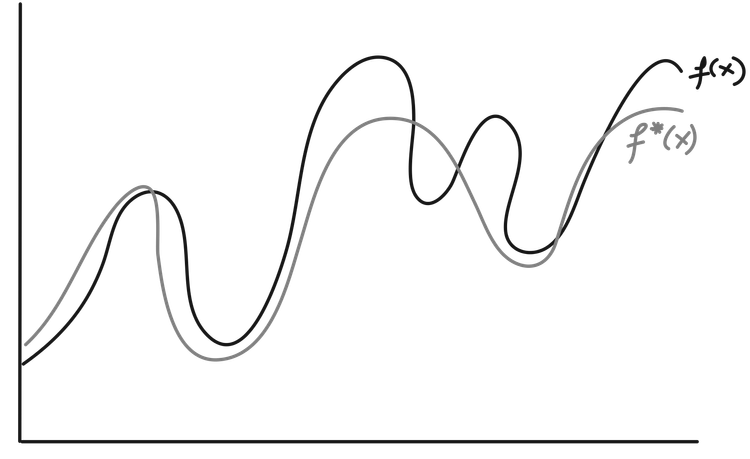The number of nodes in your input and output layers are predetermined. Your input layer has one node for each variable (or feature, as we often call them in machine learning), and the output layer has one node for every number you want to predict. But when you start adding more layers – called hidden layers – you could choose any number of nodes. At this point in Neural Network History, it’s more of an art than a science. There’s good rules of thumb for how many nodes to choose for each of our hidden layers (like slowly decreasing the number of nodes in each layer), but there’s no exact answer.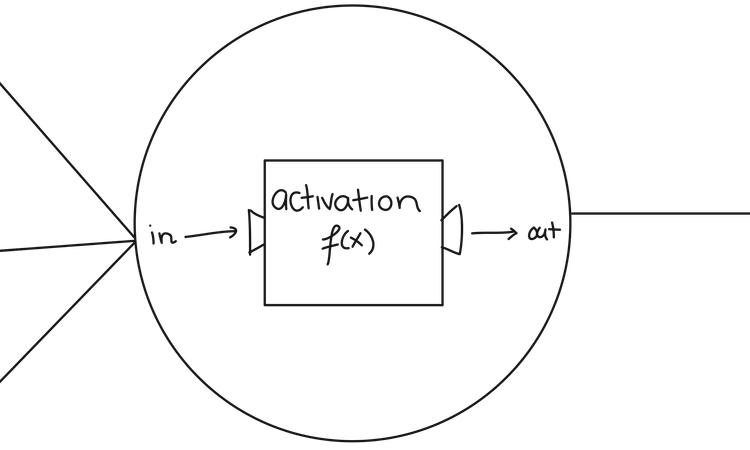Activation functions. OK, back to my comment about how some models aren’t linear (*gasp again*). Neural networks also employ activation functions in each of their nodes in order to allow the models we create to be nonlinear.

JMP Pro offers three options for activation functions: TanH, Linear, and Gaussian. In our regression model, we “secretly” were using linear activation functions. Activation functions take the input into the node and perform some kind of transformation on it before passing it along to the next layer. A linear activation (in red)  takes a value and returns it unchanged, which is exactly what we were doing in our simple regression NN above.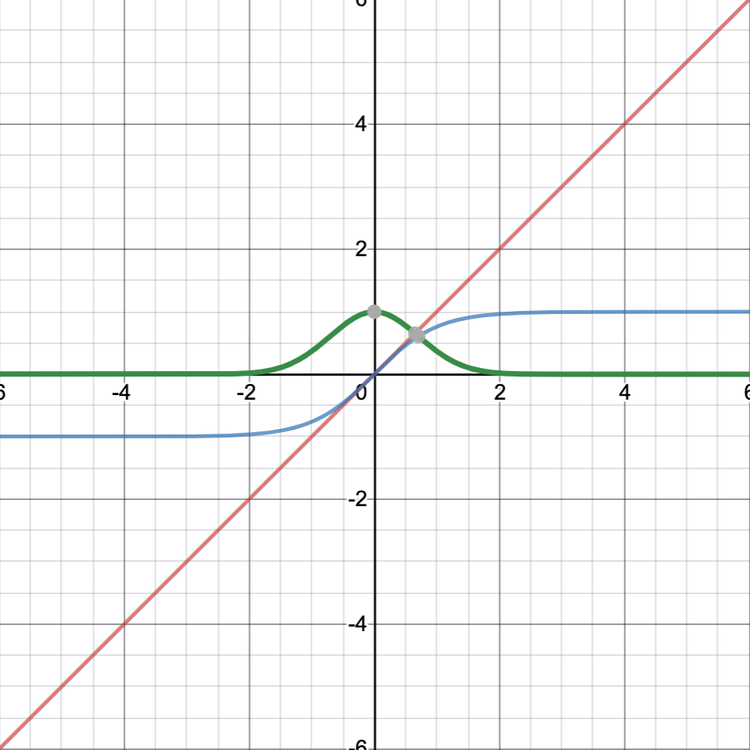But we don’t have to leave it unchanged. The TanH activation (in blue)  is one kind of Sigmoid activation (meaning it’s function looks like a stretched out S). It’s useful because it takes any input and maps it to a value between -1 and 1. Large negative values will be transformed to values close to -1, and large positive values close to 1. It’s often quite useful when your model is predicting categories (like whether someone will default on a loan or not) rather than a continuous output.

A Gaussian activation function (green) takes extreme values (either high or low) and returns values near 0. For less extreme values, it will return values close to one.

If we only use linear activations, the model we create can only be linear. But, if we use any nonlinear activation functions, we can now create nonlinear models!

JMP Pro allows you to create Neural Networks with up to 2 layers, and allows you to choose different activation functions for each layer. Using different numbers of layers, nodes, and different activation functions we can create a huge number of structures for a Neural Network. You can even use Neural Networks to predict categories rather than one continuous value.

### JMP

To build a neural network in JMP, go to Analyze>Predictive Modeling>Neural. Once the Neural platform is open, you can choose your inputs and outputs by dragging them to the X and Y boxes respectively.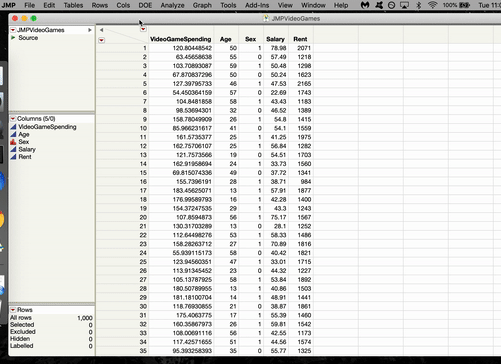This will open the Model Launch window where you can specify how many nodes you want in your two hidden layers, and which kinds of activation functions you’d like to use.

Once you click Go, JMP will build your neural network and create a table of measures of model performance (like R^2 or RMSE for continuous outputs). You can also plot the actual values by the predicted values by clicking the red triangle and selecting Plot Actual by Predicted. Similarly, we can plot our Residual values by clicking the red triangle and selecting Plot Residual by Predicted. You can also go to the red triangle menu and select Profiler to get the Prediction Profiler, which will show you more about what’s actually happening in the neural network. Black box no more!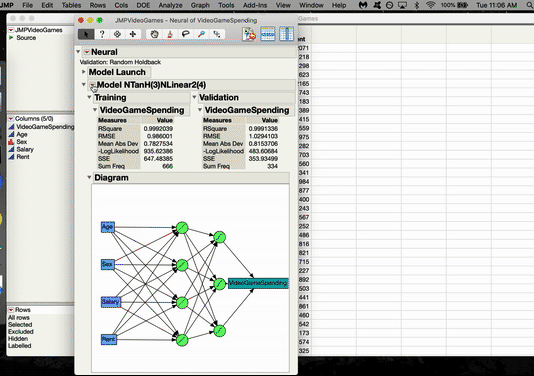### Conclusion

Neural networks are a lot of fun, and it sounds cool to tell people you’re using one. But when you look at their true nature, they’re not so different from simple linear regression. In both cases, we have to specify a structure and a learning method in order to get a working, optimized model. Neural networks do allow us to model more complex structures than simple linear regression, so they’re often useful when we think that the relationship between our inputs and our outputs is complex.

Extra layers and activation functions allow us to play around with the structure of our network, and they’re just the beginning! We can predict categorical outcomes, multiple continuous outcomes, we could have five layers, or 10! There are even more cool features we can apply to neural networks (for instance, Dropout, or other activation functions we didn’t cover here), but now that you know the basics, you can explore all these things a little more confidently.

Article Labels
Article Tags